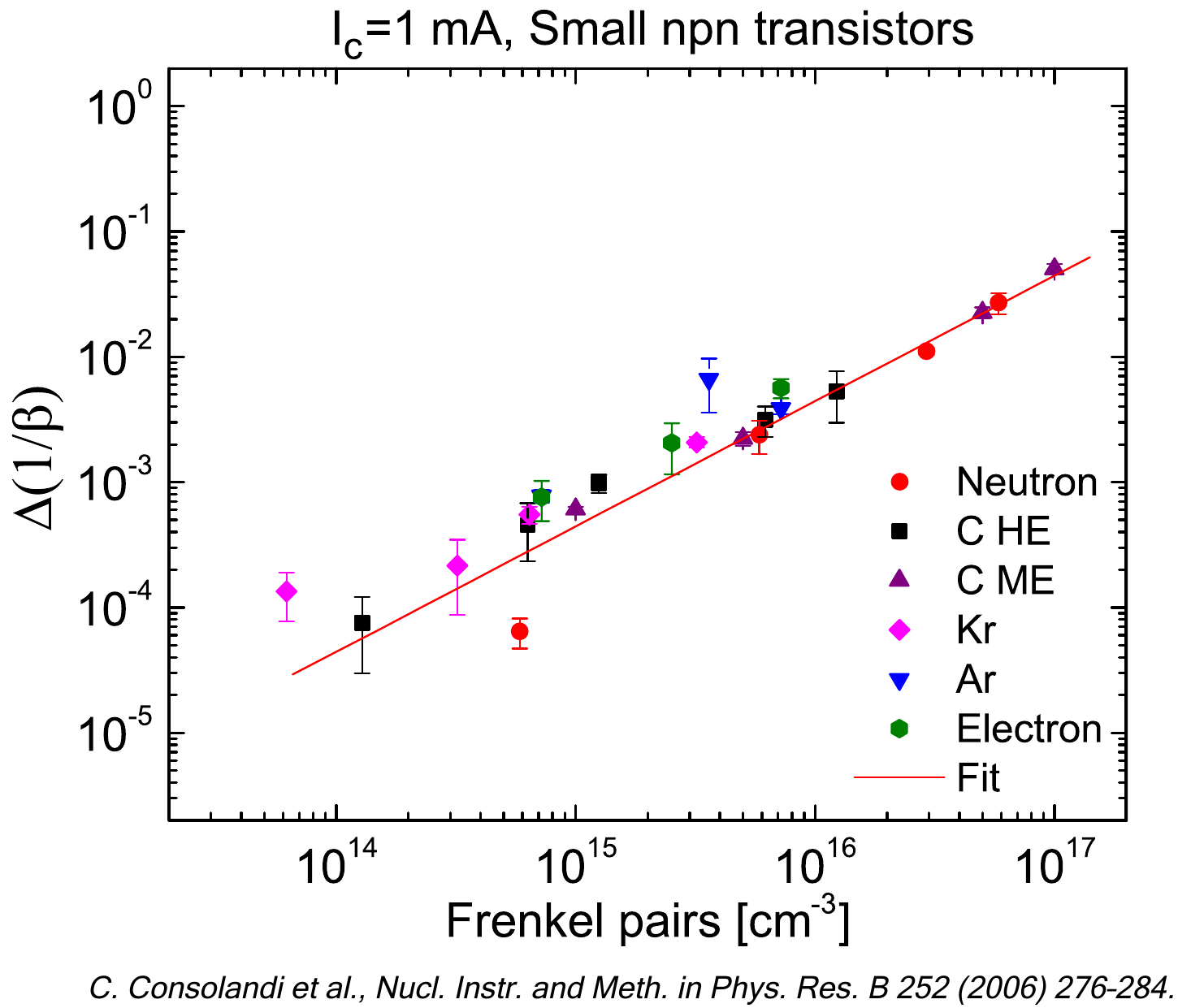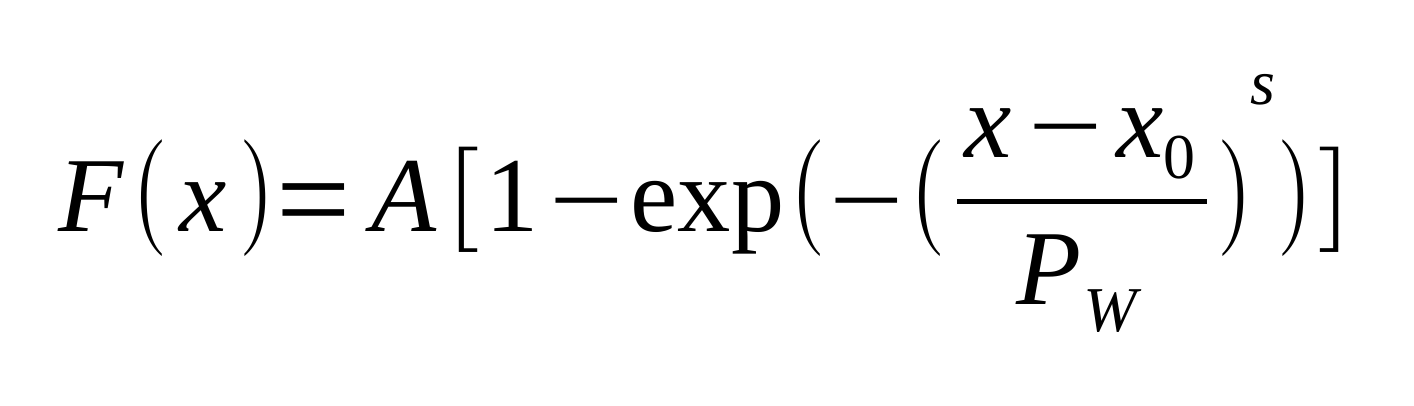SR-NIEL – 7 Screened Relativistic (SR) Treatment for NIEL Dose Nuclear and Electronic Stopping Power Calculator (version 10.11)The occurrence probability of events due to large energy depositions in a device - like those commonly referred to as single event effects (SEE) – is usually expressed by means of the cross section as a function of LET – or mass electronic stopping power or mass restricted energy loss - of the device material (e.g., see Sects. 11.4-11.4.9 of [Leroy and Rancoita (2016)] and references therein).

For particles impinging perpendicularly to the device front face, the functional form for expressing the SEE cross section for a device is commonly provided by the Weibull function. Such a cross section is obtained using measurements with uniform monodirectional particle fluences. The distribution of particle paths inside the device is expected and is found to follow that expressed by Eq. (1) of [Luke and Buehler (1998)], as discussed in [ECSS-E-HB-10-12A] and [ECSS‐E‐ST‐10‐12C].

The current calculator allows one to determine the equivalent SEE cross section when the same device is exposed to isotropically distributed particles like, for instance, to cosmic rays in space missions. The equivalent cross section is obtained by means of a GEANT4 simulation.

The following link allows one to access to the determination of the device cross section due to a large energy deposition (e.g., SEE cross section) caused by isotropically distributed particles:

### How to use the present calculator for SEE cross section for isotropic particles fields

This tool allows one to determine the SEE cross section for devices exposed to isotropically distributed particle from a SEE cross section expressed by a Weibull function obtained using measurements with perpendicularly impinging particles on device front face.

The input parameters and options for the tool are described below. When the input form has been completed, pressing the "CALCULATE" button will start the calculation and open the "Results" page (allow for pop-up in your browser settings). The result page will be also linked at the bottom of the calculator page.

### Input Parameters:

- Device dimensions
- SEE cross section (Weibull function parameters)

### Device dimension

The user has to set the device dimensions: heigh (h), width (b) and depth (t) in μm. See the following figure for target device geometry.### SEE cross section

This section define parameters that are included in the Weibull function:where x is the stopping power (or the restricted energy loss) in MeV cm2 mg-1A is the saturation cross section, x0 is the stopping power (or LET) threshold parameter, PW is the width parameter and s is a dimensionless exponent.

### Result

The result page contains - as a function of the traversed path inside the device - both the probability of particles travelling through the sensitive region with a pathlength d and the integral probability for a pathlength larger than d. The input cross section as function of the stopping power (or LET) plotted along with the calculated isotropic cross section. The table reports the isotropic cross section values as a function of the stopping power.

This data file can be downloaded by means of the link at the top of the table and, eventually, used as input file for other sr-niel web-calculators.